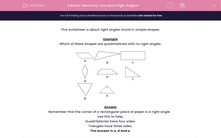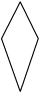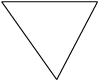# Geometry: How Many Right Angles?

In this worksheet, students state which of the quadrilaterals and triangles shown have right angles (angles of 90 degrees).Key stage:  KS 2

Curriculum topic:   Maths and Numerical Reasoning

Curriculum subtopic:   2D Shapes: Triangles, Quadrilaterals and Polygons

Difficulty level:#### Worksheet Overview

This worksheet is about right angles found in simple shapes.

Example

Which of these shapes are quadrilaterals with no right angles.abcdefgh

Remember that the corner of a rectangular piece of paper is a right angle.

Use this to help.